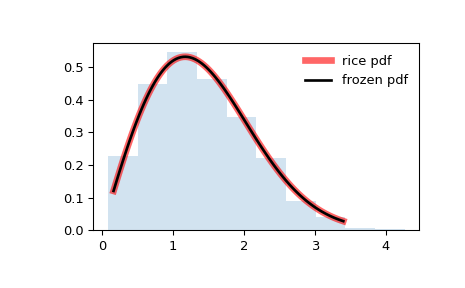# scipy.stats.rice¶

scipy.stats.rice(*args, **kwds) = <scipy.stats._continuous_distns.rice_gen object>[source]

A Rice continuous random variable.

As an instance of the rv_continuous class, rice object inherits from it a collection of generic methods (see below for the full list), and completes them with details specific for this particular distribution.

Notes

The probability density function for rice is:

$f(x, b) = x \exp(- \frac{x^2 + b^2}{2}) I_0(x b)$

for $$x >= 0$$, $$b > 0$$. $$I_0$$ is the modified Bessel function of order zero (scipy.special.i0).

rice takes b as a shape parameter for $$b$$.

The probability density above is defined in the “standardized” form. To shift and/or scale the distribution use the loc and scale parameters. Specifically, rice.pdf(x, b, loc, scale) is identically equivalent to rice.pdf(y, b) / scale with y = (x - loc) / scale.

The Rice distribution describes the length, $$r$$, of a 2-D vector with components $$(U+u, V+v)$$, where $$U, V$$ are constant, $$u, v$$ are independent Gaussian random variables with standard deviation $$s$$. Let $$R = \sqrt{U^2 + V^2}$$. Then the pdf of $$r$$ is rice.pdf(x, R/s, scale=s).

Examples

>>> from scipy.stats import rice
>>> import matplotlib.pyplot as plt
>>> fig, ax = plt.subplots(1, 1)


Calculate a few first moments:

>>> b = 0.775
>>> mean, var, skew, kurt = rice.stats(b, moments='mvsk')


Display the probability density function (pdf):

>>> x = np.linspace(rice.ppf(0.01, b),
...                 rice.ppf(0.99, b), 100)
>>> ax.plot(x, rice.pdf(x, b),
...        'r-', lw=5, alpha=0.6, label='rice pdf')


Alternatively, the distribution object can be called (as a function) to fix the shape, location and scale parameters. This returns a “frozen” RV object holding the given parameters fixed.

Freeze the distribution and display the frozen pdf:

>>> rv = rice(b)
>>> ax.plot(x, rv.pdf(x), 'k-', lw=2, label='frozen pdf')


Check accuracy of cdf and ppf:

>>> vals = rice.ppf([0.001, 0.5, 0.999], b)
>>> np.allclose([0.001, 0.5, 0.999], rice.cdf(vals, b))
True


Generate random numbers:

>>> r = rice.rvs(b, size=1000)


And compare the histogram:

>>> ax.hist(r, density=True, histtype='stepfilled', alpha=0.2)
>>> ax.legend(loc='best', frameon=False)
>>> plt.show()Methods

 rvs(b, loc=0, scale=1, size=1, random_state=None) Random variates. pdf(x, b, loc=0, scale=1) Probability density function. logpdf(x, b, loc=0, scale=1) Log of the probability density function. cdf(x, b, loc=0, scale=1) Cumulative distribution function. logcdf(x, b, loc=0, scale=1) Log of the cumulative distribution function. sf(x, b, loc=0, scale=1) Survival function (also defined as 1 - cdf, but sf is sometimes more accurate). logsf(x, b, loc=0, scale=1) Log of the survival function. ppf(q, b, loc=0, scale=1) Percent point function (inverse of cdf — percentiles). isf(q, b, loc=0, scale=1) Inverse survival function (inverse of sf). moment(n, b, loc=0, scale=1) Non-central moment of order n stats(b, loc=0, scale=1, moments=’mv’) Mean(‘m’), variance(‘v’), skew(‘s’), and/or kurtosis(‘k’). entropy(b, loc=0, scale=1) (Differential) entropy of the RV. fit(data) Parameter estimates for generic data. See scipy.stats.rv_continuous.fit for detailed documentation of the keyword arguments. expect(func, args=(b,), loc=0, scale=1, lb=None, ub=None, conditional=False, **kwds) Expected value of a function (of one argument) with respect to the distribution. median(b, loc=0, scale=1) Median of the distribution. mean(b, loc=0, scale=1) Mean of the distribution. var(b, loc=0, scale=1) Variance of the distribution. std(b, loc=0, scale=1) Standard deviation of the distribution. interval(alpha, b, loc=0, scale=1) Endpoints of the range that contains alpha percent of the distribution

#### Previous topic

scipy.stats.rayleigh

#### Next topic

scipy.stats.recipinvgauss